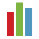6852 views
Daily Levels to measure Volatility in Crypto. Free Indicator.
Invite-only script

Access to this script is restricted to users authorized by the author and usually requires payment. You can add it to your favorites, but you will only be able to use it after requesting permission and obtaining it from its author. Contact CryptoVolatility for more information, or follow the author's instructions below.

TradingView does not suggest paying for a script and using it until you 100% trust its author and understand how the script works. In many cases you can find a good open-source alternative for free in our Public Library.

Want to use this script on a chart?raikrau
@raikrau, I can no longer manage access to the indicator with just a 'Pro+' subscription, so here is the code for everyone, just open the Pine Script Editor, paste it in, save it and add to your chart. Good luck :)

// Global settings
study(title = "DailyATR", overlay = true)

// Indicator input parameters:
// - ATR moving average period for ATR smoothness;
atrMAPeriod = input(14)
// - ATR timeframe to be used. Allowed values are (case sensitive!) 'D' for daily, 'W' for weekly, 'M' for monthly.
// Others values are also possible, but not tested.
// Note: there's seems to be a bug in Pine Script that not allows to use a preferrable form:
// atrTimeframe = input(title = "ATR timeframe", defval = 'D', options = )
atrTimeframe = input(title = "ATR timeframe", defval = 'D')
//atrTimeframe = input('D', type = resolution)
atrMultiplier = input(title = "ATR multiplier", defval = 1.0)

// ATR of needed period and timeframe
tfATR = security(tickerid, atrTimeframe, atr(atrMAPeriod))

// Open, High, Low and Time of needed period accordingly
tfOpen = security(tickerid, atrTimeframe, open)
tfHigh = security(tickerid, atrTimeframe, high)
tfLow = security(tickerid, atrTimeframe, low)
tfTime = security(tickerid, atrTimeframe, time)
// tfOpen in a new period start bar and NaN in any other bar
tfStart = (change(tfTime) != 0) ? tfOpen : na

// Upper and lower lines
upper = tfATR * atrMultiplier + tfLow
lower = tfHigh - tfATR * atrMultiplier

// Data from a dayly chart - to define where days start
//dayOpen = security(tickerid, 'D', open)
//dayTime = security(tickerid, 'D', time)
// dayOpen in a day start bar and NaN in any other bar
//dayStart = (change(dayTime) != 0) ? dayOpen : na

//www.cryptovolatility.net
// Maximal and minimal price from the period start
maximumClose = na(tfStart) ? max(maximumClose, close) : close
minimumClose = na(tfStart) ? min(minimumClose, close) : close

// Line colors calculation - transparency depends on line broken or not
upperColor = (maximumClose > upper) ? color(green, 0) : color(green, 0)
lowerColor = (minimumClose < lower) ? color(red, 0) : color(red, 0)

// Upper and lower lines draw
plot(upper, color = upperColor, linewidth = 1, title = "High", style = circles, join = false, editable = true)
plot(lower, color = lowerColor, linewidth = 1, title = "Low", style = circles, join = false, editable = true)
// Period open price draw
plot(tfOpen, color = black, linewidth = 1, title = "Day Open", style = circles, join = false, editable = true)

//plotshape(dayStart, text = "test", color = black, size=size.tiny, location = location.abovebar, style = shape.circle, textcolor = black)

//barsBack = na(dayStart) ? barsBack + 1 : 0
//plot(barsBack, color = green, linewidth = 2, style = line)

//www.cryptovolatility.net
//plot(maximumClose, color = red, linewidth = 2, style = line)
//plot(minimumClose, color = green, linewidth = 2, style = line)